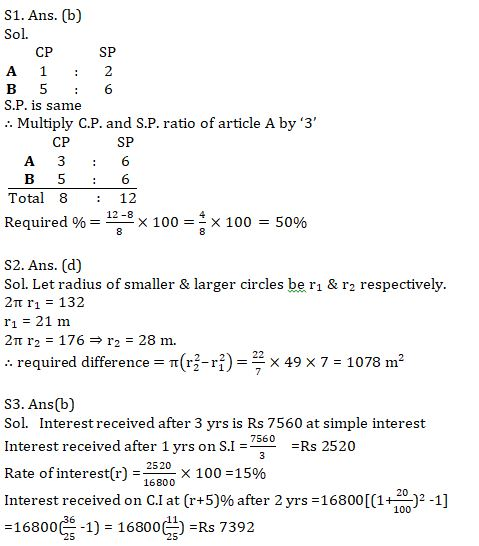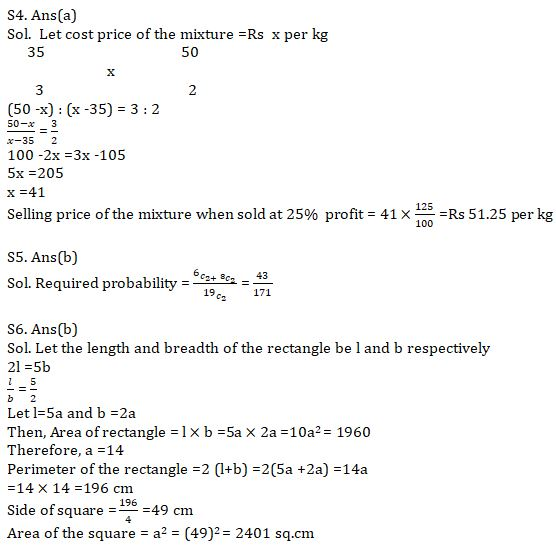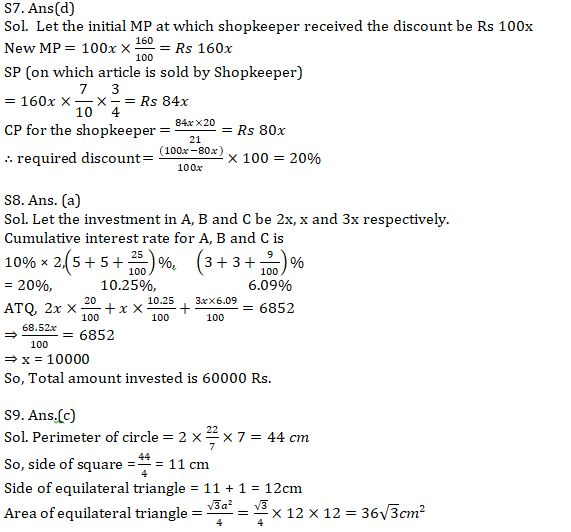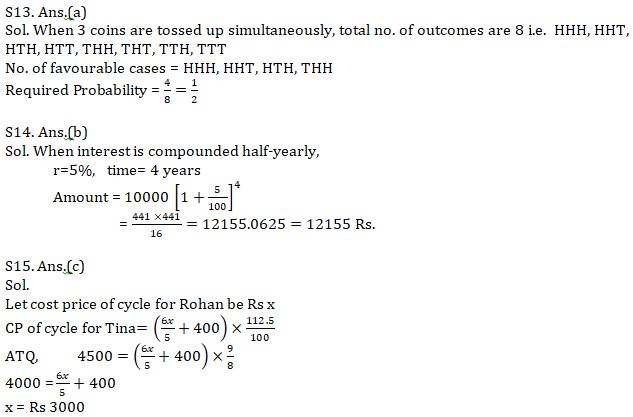Latest Banking jobs   »   Quantitative Aptitude Quiz For IBPS Clerk...

# Quantitative Aptitude Quiz For IBPS Clerk Prelims 2021- 8th August

Q1. Man sold article A at 100% profit and article B at 20% profit. If selling price of article A and article B is same then find his overall profit percentage
(a) 40%
(b) 50%
(c) 55%
(d) 60%
(e) 75%

Q2. The circumference of two circles is 132 m and 176 m respectively. What is difference between the area of larger circle and area of smaller circle ? (in m²)
(a) 1052
(b) 1128
(c) 1258
(d) 1078
(e) 1528

Q3.Aakash invested Rs 16800 on simple interest at r % p.a. for 3 yrs and received Rs 7560 as total interest. Find the interest amount received by Aakash if the same amount is invested on compound interest at (r+5)% rate of interest after 2 yrs?
(a) Rs 7560
(b) Rs 7392
(c) Rs 7120
(d) Rs 7820
(e) Rs 7460

Q4.Type A milk of cost price Rs 35 per litre is mixed with Type B milk having cost price rs 50 per litre in the ratio 3:2 respectively, then find the selling price(per kg) of the final mixture when sold at 25% profit ?(in rs)
(a) 51.25
(b) 48.75
(c) 53.3
(d) 57.4
(e) 53.5

Q5.A bag contains 6 Blue balls, 5 Red balls and 8 yellow balls. If 2 balls are taken out from the bag randomly, then find the probability of both balls being of blue colour or yellow colour?
(a) 41/171
(b) 43/171
(c) 37/169
(d) 43/169
(e) 47/143

Q6. Two times the length of a rectangle is equal to 5 times of its breadth. The area of the rectangle is 1960 sq.cm, then find what is the area of a square, if the perimeter of which is equal to that of a rectangle.
(a) 2304 sq.cm
(b) 2401 sq.cm
(c) 2500 sq.cm
(d) 2209 sq.cm
(e) 2025 sq.cm

Q7. A shopkeeper purchased an article at some discount on marked price. He raised the initial marked price of an article by 60%. Then he allows two successive discount of 30% and 25% on it. On selling the article, he still made a profit of 5% on it. Find at what discount percentage the shopkeeper has bought the article?
(a) 40%
(b) 35%
(c) 30%
(d) 20%
(e) 25%

Q8. A man invested Rs.P in three different schemes – A, B and C in the ratio 2 : 1 : 3 respectively. He invested in scheme A at the rate of 10% p.a. at SI for 2 years, in scheme B at the rate of 5% p.a at C.I compounded annually for 2 years and in scheme C at the rate of 6% p.a at CI compounded half yearly for 1 year and received total interest of Rs. 6852. Find the value of P.
(a) Rs.60000
(b) Rs.72000
(c) Rs.48000
(d) Rs.120000
(e) can’t be determined.

Q9. Perimeter of a circle and a square are same and radius of circle is 7 cm. Find the area of equilateral triangle whose side is 1 cm more than the side of square?
(a) 48 √3 cm²
(b) 54√3 cm²
(c) 36 √3 cm²
(d) 24 √3 cm²
(e) 12 √3 cm²

Q10. There are 6 Blue balls and 4 Red balls in a bag. If three balls are drawn from the bag, then find the probability of getting atleast 1 Red ball?
(a) 1/6
(b) 1/2
(c) 5/6
(d) 4/5
(e) 2/5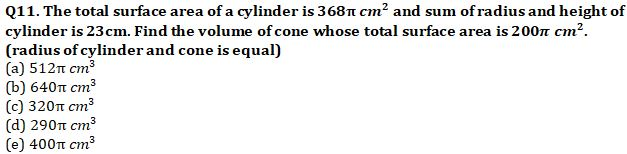Q12. CP of 10 article is equal to SP of 8 article. What percent of discount is given if MRP is 100% more than CP?
(a) 20%
(b) 37.5%
(c) 33.33%
(d) 25%
(e) none of

Q13. 3 coins are tossed up simultaneously. Find the probability of getting atleast 2 heads ?
(a)1/2
(b) 3/8
(c) 5/8
(d) 3/4
(e) 1/4

Q14. A bank offers an interest of 10% per annum which is compounded half yearly. Krishan invests Rs.10000, what is the total amount he will earn after 2 years? (approximately)
(a) Rs 14,641
(b) Rs 12,155
(c) Rs 11,011
(d) Rs 13,000
(e) Rs 13,310

Q15. Rohan sells his cycle to Mohan at 20% profit and Mohan spends Rs 400 on the repairing of cycle after that he sold it to Tina at 12.5% profit. If the cost price of cycle for Tina is Rs 4500, then find the cost price of cycle for Rohan?
(a) Rs 2500
(b) Rs 3500
(c) Rs 3000
(d) Rs 4200
(e) Rs 4000

Solution